# Thermodynamics¶

This module calculates the instantaneous values of thermodynamic state variables for the particles of a given group.

class halmd.observables.thermodynamics(args)

Construct thermodynamics module.

Parameters: args (table) – keyword arguments args.group – instance of halmd.mdsim.particle_groups args.box – instance of halmd.mdsim.box
particle_number()

Returns the number of particles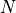selected by args.group.

density()

Returns the number density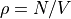using the volume from args.box.

kinetic_energy()

Returns the mean kinetic energy per particle: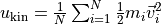.

potential_energy()

Returns the mean potential energy per particle:, where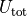denotes the sum of external and pair potentials.

internal_energy()

Returns the mean internal energy per particle: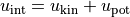.

pressure()

Returns the pressure computed from the virial: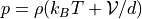.

temperature()

Returns the instantaneous temperature as given by the kinetic energy: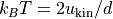.

total_force()

Returns the total force: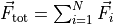.

center_of_mass_velocity()

Returns the centre-of-mass velocity: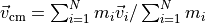.

center_of_mass()

Returns the centre of mass: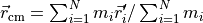, where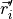refers to absolute particle positions, i.e., extended by their image vectors for periodic boundary conditions.

mean_mass()

Returns the mean particle mass: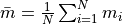.

virial()

Returns mean virial per particle as computed from the trace of the potential part of the stress tensor: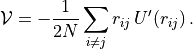stress_tensor()

Returns the elements of the stress tensor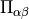as a vector. The first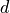(= dimension) elements contain the diagonal followed byoff-diagonal elements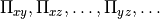The stress tensor is computed as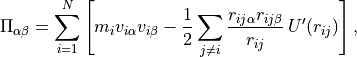where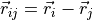in nearest image convention.

dimension

Space dimensionof the simulation box as a number.

group

Instance of halmd.mdsim.particle_groups used to construct the module.

writer(args)

Write state variables to a file.

Parameters: args (table) – keyword arguments args.file – instance of file writer args.every (number) – sampling interval args.location (string table) – location within file (optional) args.fields (table) – data fields to be written (optional) instance of group writer

The optional argument location specifies a path in a structured file format like H5MD given as a table of strings. It defaults to {"observables", group.global and nil or group.label}.

The optional table fields specifies which data fields are written. It may either be passed as an indexed table, e.g. {"pressure"}, or as a dictionary, e.g., {p = "pressure"}; the table form is interpreted as {pressure = "pressure", …}. The keys denote the field names in the file and are appended to location. The values specify the data methods of the thermodynamics module, i.e., all methods described above except for dimension and group. The default is {"potential_energy", "pressure", "temperature", "center_of_mass_velocity"}.

disconnect()

Disconnect thermodynamics writer from observables sampler.